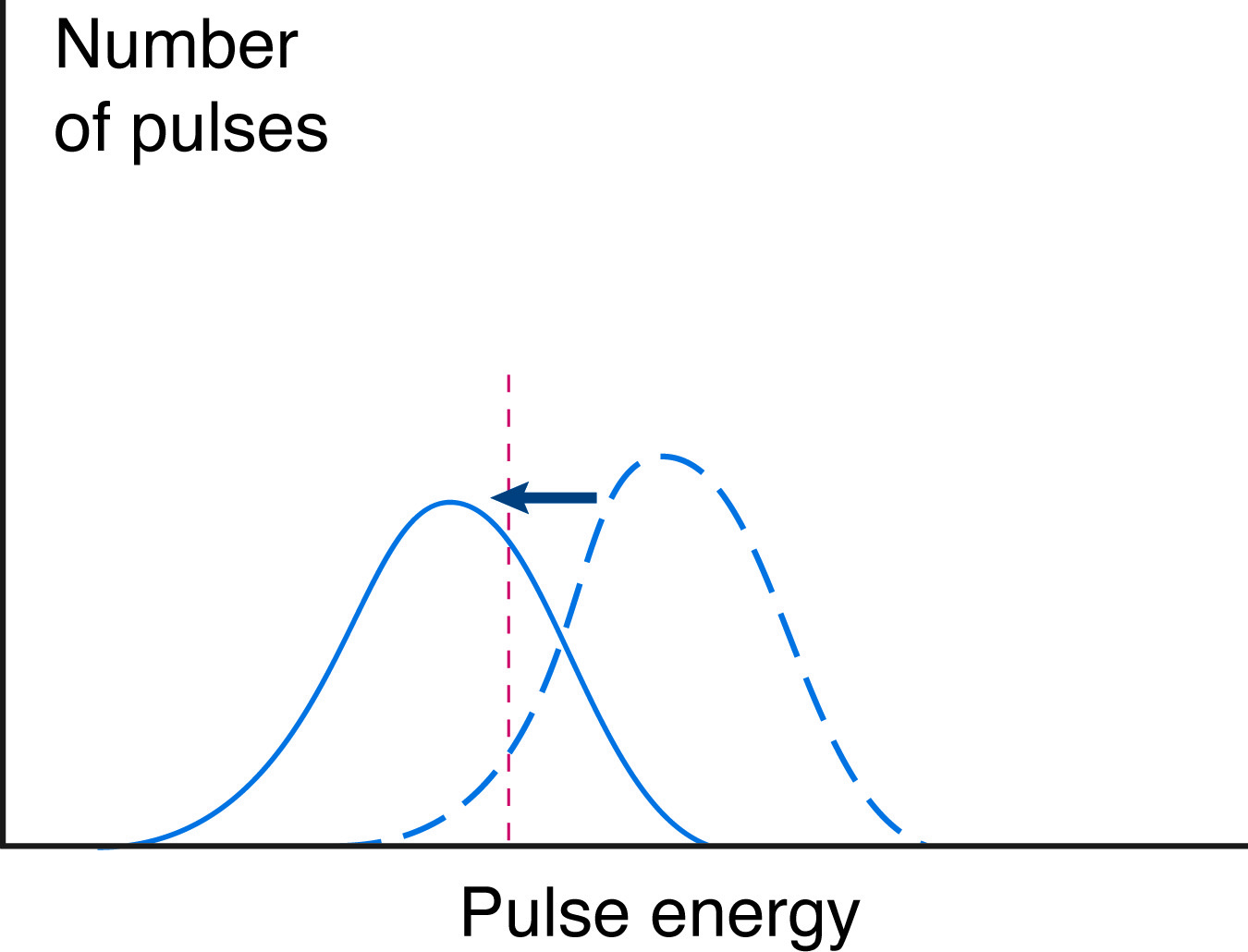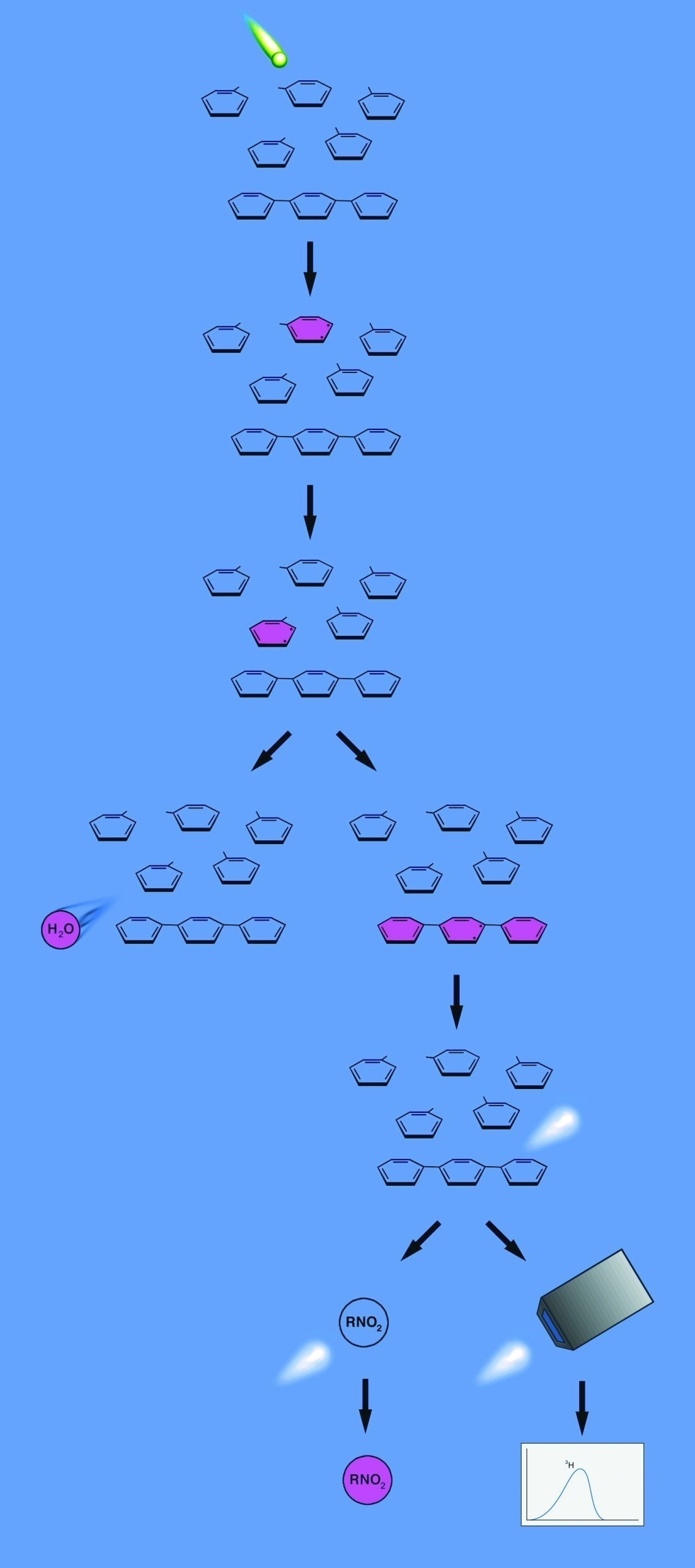## Counting Efficiency and Quenching

While the effectiveness of a scintillation cocktail may be expressed a number of ways, it is most often given as the percentage of emission events that produce a detectable pulse of photons, referred to as the counting efficiency. In other words, one way to express the counting efficiency is to take the ratio of the counts per minute to disintegrations per minute DPM expressed as a percentage (CPM/DPM). Counting efficiency varies for different isotopes, sample compositions and scintillation counters. Poor counting efficiency can be caused by an extremely low energy to light conversion rate, (scintillation efficiency) which, even optimally, will be a small value.

It has been calculated that only some 4% of the energy from a β emission event is converted to light by even the most efficient scintillation cocktails. Fortunately, this number does not vary greatly across a wide range of β-energies, which avoids an additional level of complexity in signal interpretation. However, the low efficiency in energy conversion means that low energy β particles will only generate a few photons. 3H, for example, has a maximum β energy of 0.019 MeV, which at 4% scintillation efficiency will generate about 240 photons.

The average emission energy is generally 30-40% of Emax, which would give 70-100 photons in this case. Most phototubes used in scintillation detection only detect 1 in 4 photons, so the average 3H β-emission event will produce only a 20-25 photon pulse in the counter. Clearly many emissions of below average energy, or emissions which lose photons due to sample characteristics, will fall below the level of a 1 photon event and will not register as a count on the instrument. The loss of CPM due to absorption of β-energy or photons by sample components is known as quenching. Quenching can easily reduce pulses below the detection limit of the counter, thus reducing the overall counting efficiency.

### Quenching

Quenching is the loss of counts due to sample or cocktail characteristics. Quenching may result from a variety of components in a sample. Quenchers are customarily divided into the categories of chemical quenchers or color quenchers.

Chemical quenchers absorb radioactive energy before it is converted to light. Therefore, chemical quenchers reduce the number of photons generated by each β-particle. Color quenchers absorb light in the range of the wavelength emitted by the scintillator. In this case the number of photons emitted is not changed, but the number reaching the photomultiplier tube is reduced.Strong quenching can shift the majority of pulses below the threshold of detection (marked by the dashed red line).

In both types of quenching, the energy of all light pulses is reduced, and the total CPM is reduced by the number of pulses quenched to below detectable levels. This leads to an underestimate of the total counts, and thus of the isotope present. It also leads to an apparent shift in the energy spectrum of the sample.Chemical quenching and color quenching can keep energy from a radioactive event from making its way through the scintillation mechanism to the photomultiplier tube. The stylized diagram above presents chemical quenching by water and color quenching by an organic nitrate as two possible obstacles to efficient counting.

### Quench Correction

Various methods are available for quench correction. The most straightforward, but most laborious, is the use of an internal standard. A known amount of radioactivity, added to an unknown sample, will increase the DPM by a predictable amount. The difference between the increase in DPM observed and that expected is due to quenching, and allows the determination of counting efficiency for that sample. The drawback to the use of internal standards is that each sample must be counted twice. It is also inconvenient to add an internal standard to many vials.

Many scintillation counters offer the use of an external standard to correct for quenching. After initial counting, a strong γ emission source is placed next to the vial and the sample is counted again. The γ rays cause secondary emission of Compton electrons, which scintillate in the cocktail like β particles. The counts due to sample radioactivity are subtracted, leaving only the Compton electron counts. The theoretical energy distribution of the Compton electrons is compared with the measured energy spectrum to determine the extent of quenching. The samples must still be counted twice, but nothing need be added to the vials, and the process may be carried out automatically by the counter.

The analysis of the energy spectrum is commonly done by computing a "channels ratio". The detected counts are divided into channels based on their relative energies, and the number of high energy counts (with two channels, the "B" channel) is compared to the number of low energy counts (channel "A"). The ratio, calculated as B/A or B/(B+A), will change if the sample is quenched. Quenching reduces the intensity of each light pulse, so counts will appear to be of lower energy. This will shift counts from high to low energy channels, and decrease the channels ratio.

In practice, a set of quenched standards is created by adding a quenching agent to reduce the CPM of an internal standard. The channels ratio of the external standard is then determined, and a correlation is established between quench and channels ratio. The channels ratio analysis may also be applied to the sample itself to determine quenching. Again, a set of quenched standards is assembled, and a known amount of radioactivity is added to each. A curve is constructed, relating CPM/DPM to B/(B+A). Once this curve has been generated, the quench of any subsequent sample can be determined from its channels ratio. This quenching factor is then used to correct CPM to DPM.

NEXT TOPIC: The Complete Scintillation Cocktail